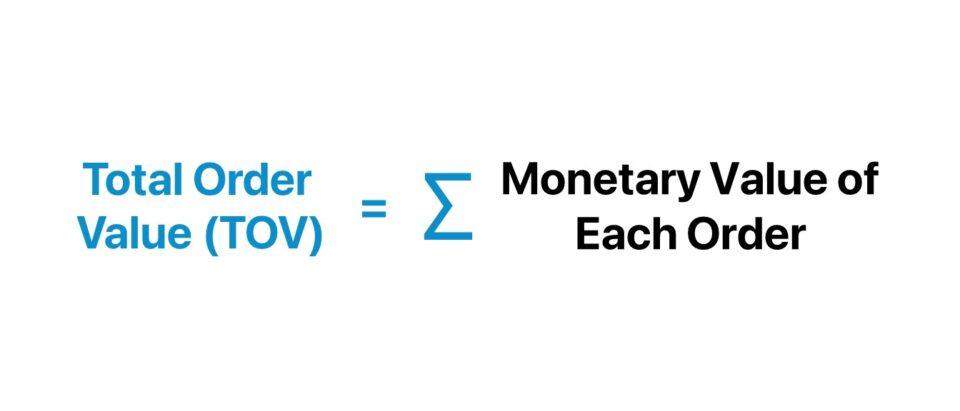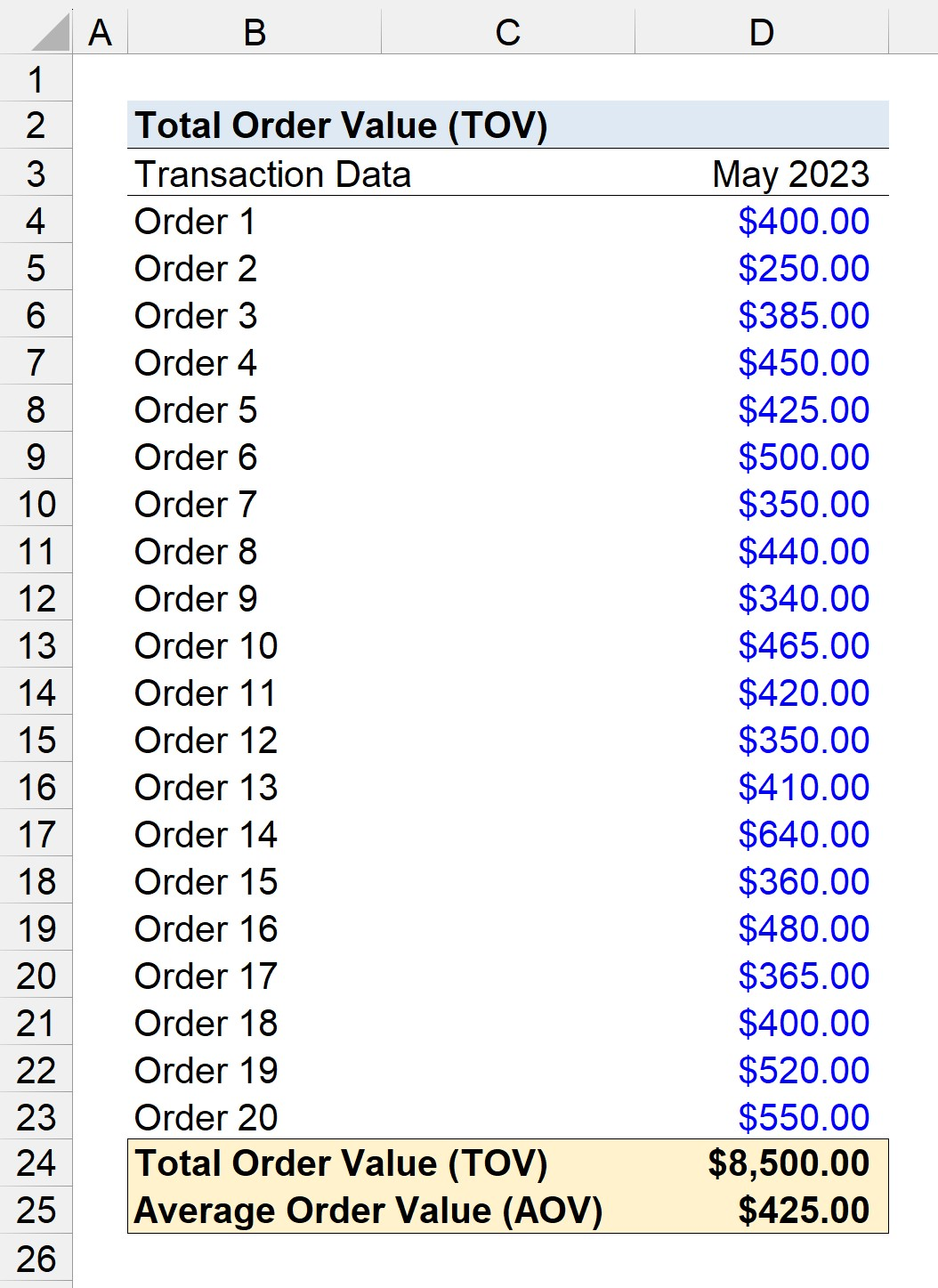Welcome to Wall Street Prep! Use code at checkout for 15% off.# Total Order Value (TOV)

Guide to Understanding Total Order Value (TOV)## How to Calculate Total Order Value?

The total order value (TOV), or “gross order value”, measures the cumulative value of all customer orders across a given time period.

The total order value is inclusive of all completed orders, without any deductions for returns, cancellations, or discounts.

By tracking the total order value (TOV), a company can obtain insights regarding its revenue trajectory and identify trends in customer spending over time.

The sum of the value of all orders placed within a stated time period must be determined to calculate the total order value (TOV).

1. Determine Periodicity → Identify the specific time frame to analyze.
2. Record Monetary Value of Orders → For each order placed in the selected period, record the total monetary value that the customer paid in all transactions, inclusive of the taxes, payment processing fees, and shipping costs incurred by customers.
3. Calculate Sum of Transaction Values → The total value of all the monetary values tied to all orders reflects the total order value (TOV).

Because the total order value (TOV) is a gross figure, without any adjustments for the incurred costs to deliver the goods or services, it is used to help understand the revenue growth of the company, as opposed to profitability.

The company can also segment customers by order value to grasp the spending patterns of their end market, e.g. the traits shared by customers with higher order values.

Further, the effectiveness of a company’s strategies to incentivize greater spending by customers, such as upselling and cross-selling strategies, can be monitored through the trends in its total order value (TOV).

• Upselling → The tactics to convince existing customers to upgrade to different products or switch to an adjacent plan with higher pricing
• Cross-Selling → The tactics related to offering complimentary or closely related products to customers that made a purchase in the past (or is right on the verge of completing a transaction).

## Total Order Value Formula (TOV)

The total order value (TOV) formula is straightforward, as it is the sum of the monetary values of all customer orders over a pre-specified period.

Total Order Value (TOV) = Σ Monetary Value of Each Order

For example, if an online retailer had 50 orders in a day — of which thirty transactions were \$40, while the remaining twenty amounted to \$50 — the total order value comes out to \$220.

• Total Order Value (TOV) = (\$40 × 30) + (\$50 × 20) = \$2,200

## What is the Difference Between TOV vs. AOV?

The total order value (TOV) measures the total value of all orders across a chosen period, while the average order value (AOV) estimates the average amount spent per order by customers.

• Total Order Value (TOV) → The TOV is the total monetary value of all orders placed within a specific period, i.e. the sum of the values of all customer orders.
• Average Order Value (AOV) → On the other hand, the AOV is the average spending of customers per transaction. The AOV is determined by dividing the total revenue by the number of customer orders. Monitoring the AOV provides insights into the purchase behavior of customers, which can be utilized to set internal sales targets and gauge the effectiveness of the current sales and marketing strategies (S&M).

The formula to compute the average order value (AOV) is as follows.

Average Order Value (AOV) = Total Order Revenue ÷ Total Number of Transactions

## Total Order Value Calculator (TOV)

We’ll now move to a modeling exercise, which you can access by filling out the form below.#### Get the Excel Template!Submitting...

## eCommerce Total Order Value Calculation Example (TOV)

Suppose an eCommerce retailer is calculating its total order value (TOV) for the month of May 2023 given the following transaction data.

Customer Transaction Data

• Order 1 = \$400.00
• Order 2 = \$250.00
• Order 3 = \$385.00
• Order 4 = \$450.00
• Order 5 = \$425.00
• Order 6 =\$500.00
• Order 7 = \$350.00
• Order 8 = \$440.00
• Order 9 = \$340.00
• Order 10 = \$465.00
• Order 11 = \$420.00
• Order 12 = \$350.00
• Order 13 = \$410.00
• Order 14 = \$640.00
• Order 15 = \$360.00
• Order 16 = \$480.00
• Order 17 = \$365.00
• Order 18 = \$400.00
• Order 19 = \$520.00
• Order 20 = \$550.00

The total order value (TOV) for the month of May is the sum of all the listed orders, which comes out to \$8,500. The average order value (AOV) can be derived from the total order value by dividing the TOV (\$8,500) by the total number of transactions (20 Orders), resulting in an AOV of \$425.00.

• Total Order Value (TOV) = \$8,500
• Average Order Value (AOV) = \$8,500 ÷ 20 = \$425.00### The Wharton Online and Wall Street Prep Private Equity Certificate Program

Level up your career with the world's most recognized private equity investing program. Enrollment is open for the Jan. 29 - Mar. 25 cohort.